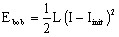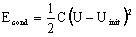# Energies and powers (electric system)

## Introduction

This section deals with the stored energy and the power dissipated in an electric system.

## Power: definition (reminder)

The instantaneous power consumed by a system with respect to two terminals (dipole) is equal to the product of the instantaneous values of the current I which passes through it and the voltage U at its terminals, respectively:

P=U.I

## Stored energy

The components that allow the storage of energy are the coils and the capacitors. The energy storage can be a temporary given that the stored energy can be then transferred via a current to the circuit that contains the component.

Component Stored energy

A coil of inductance L stores energy as magnetic energy.A capacitor of capacitance C stores energy as electric energy.## Dissipated power / Power losses by Joule effect

The components that dissipate energy are the resistive electric components (resistor / brush-segment / switch / diode).

P=U.I=R.I2